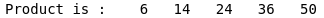# Map Function in MATLAB

• Last Updated : 22 Sep, 2021

A map function basically takes the elements of the array and applies a function to each element.  The resultant output is of the same shape as the array, but the values are the result of the function. In MATLAB, there is a similar function called arrayfun(), which we can be used to achieve the same task. The output from this arrayfunc can have any data type.

Syntax:

X = arrayfun(func,A)

Parameters:

• fun: It is a function that we are going to map on each element of the array A.
• A: It is the array on which we  are going to map the function.
• X: In variable X the output is going to be stored from the map function.

Here, arrayfun(funct,A) applies the function funct to the elements of array A. arrayfun then concatenates the outputs from funct into the output array X, so that for the ith element of A, X(i) = funct(A(i)).

The input argument funct is a function handle to a function that takes one input argument and returns a scalar.

Let’s take an example for a better understanding:

Example 1:

## Matlab

 `% MAP function in MatLab`` ` `% creating a dummy array ``% on which we are going to map a function``A = [1,2,3,4,5,6,7,8,9,10];`` ` `% Applying arrayfun()  ``output = arrayfun(@(x) x*2, A);`` ` `% displaying output``fprintf(``"output is :"``);``disp(output);`

Output:Applying arrayfun()

In the above function, firstly we have created an array to which we are going to map a custom function. The custom is x*2 i.e. multiply each element in the array by 2.

Then we are using arrayfun(@(x) x*2, A), lets break down this statement here arrayfun(fun,array) is the general syntax for mapping a function to the array. We are using @(x) and x*2 in the statement where x*2 is the custom function that we are applying and @(x) is acts as each element of that array A. After that, we’re storing the output to a variable and then using fprintf function we printed a string on display and using the disp function we display the output.

Example 2:

## Matlab

 `% MAP function in MatLab`` ` `% creating two dummy arrays ``% on which we are going to map a function``A = [1,2,3,4,5];``B = [6,7,8,9,10];`` ` `% Applying arrayfun()  ``output = arrayfun(@(x,y) x*y, A,B);`` ` `% displaying output``fprintf(``"Product is :"``);``disp(output);`

Output:Product to two arrays using arrayfun()

My Personal Notes arrow_drop_up